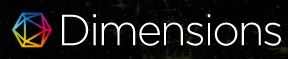### FUNGSI WRIGHT SEBAGAI SOLUSI ANALITIK PERSAMAAN DIFUSI-GELOMBANG FRAKSIONAL PADA MEDIA VISKOELASTIS

Ray Novita Yasa, Agus Yodi Gunawan

DOI: https://doi.org/10.14710/jfma.v3i1.7411

#### Abstract

A fractional diffusion-wave equations in a fractional viscoelastic media can be constructed by using equations of motion and kinematic equations of viscoelastic
material in fractional order. This article concerns the fractional diffusion-wave equations in the fractional viscoelastic media for semi-infinite regions that satisfies signalling boundary value problems. Fractional derivative was used in Caputo sense. The analytical solution of the fractional diffusion-wave equation in the fractional viscoelastic media was solved by means of Laplace transform techniques in the term of Wright function for simple form solution. For general parameters, Numerical Inverse Laplace Transforms (NILT) was used to determine the solution.

PDF

#### References

Nutting, P.G. 1921: A new general law of deformation, Journal of The Franklin Institute, Vol. 191, Edisi 5, 679-685

Blair, G. dan Reiner, M.. (1951). The rheological law underlying the Nutting equation. Flow Turbulence and Combustion - Flow Turbul Combust. 2. 225-234. 10.1007/BF00411984.

Rogosin, S. dan Mainardi, F. 2014: George William Scott Blair the pioneer of fractional calculus in rheology. Communications in Applied and Industrial Mathematics, Vol. 6, No. 1.-481; ISSN 2038-0909; DOI: 10.1685/journal.caim.481.

Nigmatullin, R. R. 1986: The realization of the generalized transfer equation in a medium with fractal geometry, Physica Status Solidi B, 133, 425430; DOI: 10.1002/pssb.2221330150.

Mainardi, F., Luchko, Y., dan Pagnini, G. 2001: The fundamental solution of the spacetime fractional diffusion equation, An International Journal for Theory and Applications, Vol. 4, No. 2, 153-192; ISSN 1311-0454.

Mainardi, F., dan Pagnini, G. 2003: The wright function as solutionsof the time-fractional diffusion equation, Applied Mathematics and Computation, Vol. 141, Edisi 1, 51-62; dx.doi.org/10.1016/S0096-3003(02)00320-X.

Povstenko, Y. 2011: Non-axisymmetric solutions to time-fractional diffusion-wave equation in an infinite cylinder, Fractional Calculus and Applied Analysis, Vol. 14, No. 3, 418-435; DOI: 10.2478/s13540-011-0026-4.

A. Bradji, A new analysis for the convergence of the gradient discretization method for multidimensional time fractional diffusion and diffusion-wave equations, Computers and Mathematics with Applications (2019),https://doi.org/10.1016/j.camwa.2019.11.001.

Y.Zheng, Z. Zhao, The time discontinuous space-time finite element methode for fractional diffusion-wave equation, Appl. Numer. Math. (2019), https://doi.org/10.1016/j.apnum.2019.09.007

Tramontana V, et al. An application of Wright functions to the photon propagation. J Quant Spectrosc Radiat Transfer (2013), http://dx.doi.org/10.1016/j.jqsrt.2013.03.008

Elsaid, A., Latief, M.S.A, Maneea, M. 2016: Similarrity Solutions for Multiterm Time- Fractional Diffusion Equation, Advances in Mathematical Physics, Hindawi Publishing Corporation, http://dx.doi.org/10.1155/2016/7304659.

Mainardi, F. 2010: Fractional calculus and wave in liear viscoelasticity, An introduction to mathematical models, ISBN-101-84816-329-0, London, Imperial College Press.

Podlubny, I. 1999: Fractional differential equation, Mathematics in Science and Engineering, Vol. 198, ISBN 0-12-558840-2, San Diego, California, Academic Press.

Wong, R. dan Zhao, Y.-Q. 1999: Smoothing of Stokes’ discontinuity for the generalized Bessel function, Proceedings of the Royal Society of London A: Mathematical, Physical

and Engineering Sciences 455, 1381-1400; DOI: 10.1098/rspa.1999.0365.

Choksi, H., dan Timol, M.G., 2014: Similarity solution for partial differential equation of fractional order, International Journal of Engineering and Innovative Technology (IJEIT), Vol. 4, Edisi 2, ISSN 2277-3754.

Sheng, H., Li, Y., dan Chen, Y.-Q. 2010: Application of numerical inverse Laplace transform algorithms in fractional calculus, Journal of The Franklin Institute, 348, 315-330;

DOI: 10.1016/j.jfranklin.2010.11.009.

Rani, D., Mishra, V., Numerical Inverse Laplace Transform based on Bernoulli Polynomials Operational Matrix for Solving Nonlinear Differential Equations, Results in Physics

(2019), doi: https://doi.org/10.1016/j.rinp.2019.102836

N.U. Khan, T. Usman and M. Aman, Some properties concerning the analysis of generalized Wright function, Journal of Computational and Applied Mathematics (2020), doi: https://doi.org/10.1016/j.cam.2020.112840.

Brancik, L., dan Smith, N. 2015: Two approaches to derive approximate formulae of NILT method with generalization, IEEE, DOI: 10.1109/MIPRO.2015.7160256.

### Refbacks

• There are currently no refbacks.

### Publisher:

Department of Mathematics, Faculty of Science and Mathematics, Diponegoro University

Mailing address: Jl. Prof Soedarto, SH, Tembalang, Semarang, Indonesia 50275

Telp./Fax             : (+6224) 70789493 / (+62224) 76480922

Website              : www.math.fsm.undip.ac.id